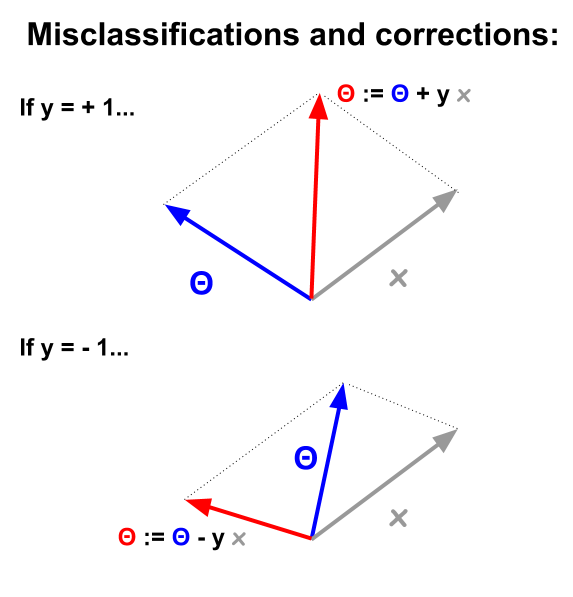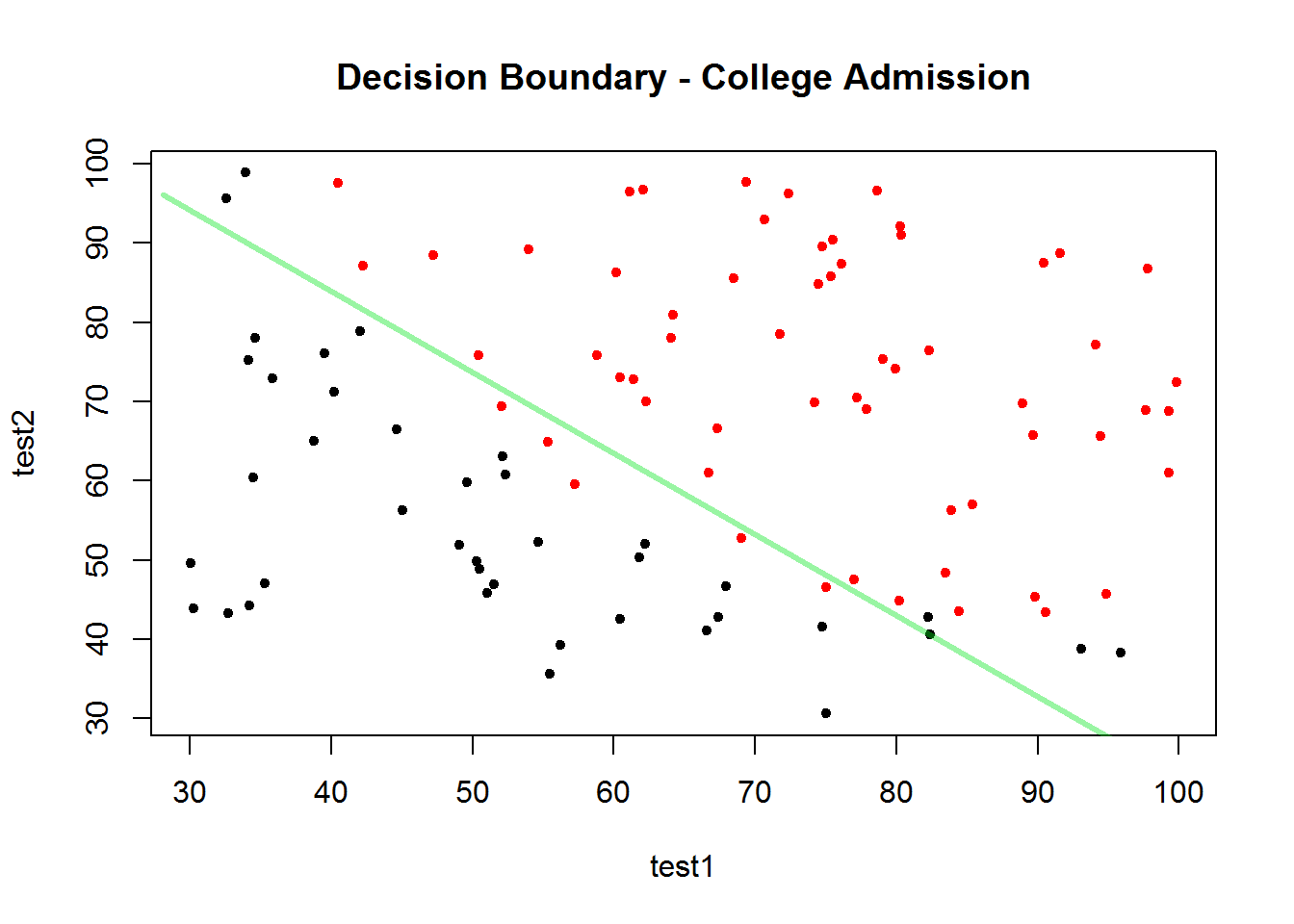### PERCEPTRON:

In machine learning, the perceptron is an algorithm for supervised learning of binary classifiers: functions that can decide whether an input (represented by a vector of numbers) belongs to one class or another (Wikipedia). The idea is similar to logistic regression, although the optimization is different:

Vectorially, the $$d$$ features or attributes of an example are $$\bf x$$, and the idea is to “pass” the example if:

$$\displaystyle \sum_{1}^d \theta_i x_i > \text{theshold}$$ or…

$$h(x) = \text{sign}\big(\displaystyle \sum_{1}^d \theta_i x_i - \text{theshold}\big)$$. The sign function results in $$1$$ or $$-1$$, as opposed to $$0$$ and $$1$$ in logistic regression.

The threshold will be absorbed into the bias coefficient, $$+ \theta_0$$. The formula is now:

$$h(x) = \text{sign}\big(\displaystyle \sum_0^d \theta_i x_i\big)$$, or vectorized:

$$h(x) = \text{sign}(\theta^T\bf x)$$.

Misclassified points will have:

$$\text{sign}(\theta^T\bf x) \neq y_n$$, meaning that the dot product of $$theta$$ and $$\bf x_n$$ will be positive (vectors in the same direction), when $$y_n$$ is negative, or the dot product will be negative (vectors in opposite directions), while $$y_n$$ is positive:The process starts with random weights or coefficients, and calculates for every misclassified points or examples $$n$$ in the training sample:

$$\color{red}{\theta} := \color{blue}{\theta} + y_n \times \bf x_n$$

In this example I use logistic regression to get a decission boundary. We are looking at two test results, and the ultimate outcome of whether the student gets into college or not.

The code is as follows:

dat = read.csv("perceptron.txt", header=F)
colnames(dat) = c("test1","test2","y")
dat[1:5,]
##      test1    test2 y
## 1 34.62366 78.02469 0
## 2 30.28671 43.89500 0
## 3 35.84741 72.90220 0
## 4 60.18260 86.30855 1
## 5 79.03274 75.34438 1
plot(test2 ~ test1, col = as.factor(y), pch = 20, data=dat,
main = "Decision Boundary - College Admission")

fit = glm(y ~ test1 + test2, family = "binomial", data = dat)
coefs = coef(fit)
x = c(min(dat[,1])-2,  max(dat[,1])+2)
y = c((-1/coefs) * (coefs * x + coefs))
lines(x, y, lwd = 3, col = rgb(0,.9,.1,.4))The boundary decision line corresponds to:

$$0 = \theta_0 + \theta_1 \times \text{test1} + \theta_2 \times \text{test2}$$. Hence, $$\text{test2}=(\frac{-1}{\theta_2})\times (\theta_0 + \theta_1 \times \text{test1} ).$$